### Hessian and CRP Jacobian Scaling

The rows and columns of the Hessian and crossproduct Jacobian matrix can be scaled when using the trust region, Newton-Raphson, double dogleg, and Levenberg-Marquardt optimization techniques. Each element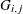,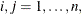is divided by the scaling factor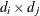, where the scaling vector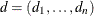is iteratively updated in a way specified by the HESCAL=option, as follows:

• No scaling is done (equivalent to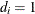).

• First iteration and each restart iteration: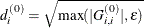• refer to Moré (1978):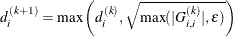• refer to Dennis, Gay, and Welsch (1981):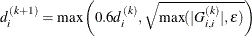•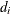is reset in each iteration: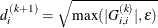whereis the relative machine precision or, equivalently, the largest double precision value that when added to 1 results in 1.